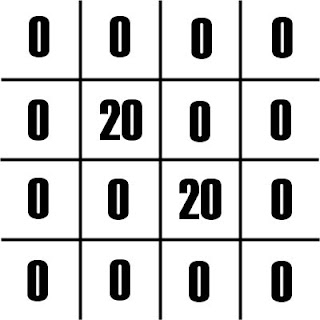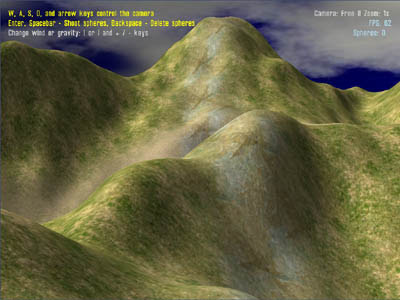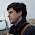## Monday, February 25, 2013

### Simple terrain smoothing

After creating or importing terrain you may find it to be too steep or jagged. Part of this depends on how your terrain system is written, but it also depends on the resolution of the terrain and terrain you're importing.

Let's image the grid below signifies sections of our terrain, with the numbers representing elevation. We can see there are two peaks that are 20 meters tall, surrounded immediately by flat terrain that is 0 meters tall. So we basically have two very steep and pointy peaks that are 20 meters high.To smooth the section represented here in blue, we total up all of the heights in the red squares (which gives us 20), and we divide by the number of red squares (there are 8 squares, so 20 / 8 = 2.5), and we average that result and the height of the blue square together. (20 + 2.5) / 2 = 11.25. We now have our new smoothed value, and we replace the blue square with that value.

Here we can see the results after smoothing out just the one square in the example above.Here's what we get when we run the algorithm against every square in the grid.

Here's the algorithm:
```public void SmoothTerrain(int Passes)
{
float[,] newHeightData;

while (Passes > 0)
{
Passes--;

// Note: MapWidth and MapHeight should be equal and power-of-two values
newHeightData = new float[MapWidth, MapHeight];

for (int x = 0; x < MapWidth; x++)
{
for (int y = 0; y < MapHeight; y++)
{
int adjacentSections = 0;
float sectionsTotal = 0.0f;

if ((x - 1) > 0) // Check to left
{
sectionsTotal += HeightData[x - 1, y];

if ((y - 1) > 0) // Check up and to the left
{
sectionsTotal += HeightData[x - 1, y - 1];
}

if ((y + 1) < MapHeight) // Check down and to the left
{
sectionsTotal += HeightData[x - 1, y + 1];
}
}

if ((x + 1) < MapWidth) // Check to right
{
sectionsTotal += HeightData[x + 1, y];

if ((y - 1) > 0) // Check up and to the right
{
sectionsTotal += HeightData[x + 1, y - 1];
}

if ((y + 1) < MapHeight) // Check down and to the right
{
sectionsTotal += HeightData[x + 1, y + 1];
}
}

if ((y - 1) > 0) // Check above
{
sectionsTotal += HeightData[x, y - 1];
}

if ((y + 1) < MapHeight) // Check below
{
sectionsTotal += HeightData[x, y + 1];
}

newHeightData[x, y] = (HeightData[x, y] + (sectionsTotal / adjacentSections)) * 0.5f;
}
}

// Overwrite the HeightData info with our new smoothed info
for (int x = 0; x < MapWidth; x++)
{
for (int y = 0; y < MapHeight; y++)
{
HeightData[x, y] = newHeightData[x, y];
}
}
}
}```Here's some purposely jagged terrain, without any smoothingTerrain after one smoothing passAfter five smoothing passesAfter 50 smoothing passes. 20 passes would probably have been fine

1.2.3.